# Preamble

The purpose of the present vignette is to demonstrate the visualisation capacities of mstate, using both base R graphics and the ggplot2 package (Wickham 2016). To do so, we will use the dataset used to illustrate competing risks analyses in Section 3 of the Tutorial by Putter, Fiocco, and Geskus (2007) . The dataset is available in mstate under the object name aidssi.

We can begin by loading both the mstate and ggplot2 libraries, and set a general theme for our plots:

# Load libraries
library(mstate)
library(ggplot2)
#> Warning: replacing previous import 'vctrs::data_frame' by 'tibble::data_frame'

# Set general ggplot2 theme
theme_set(theme_bw(base_size = 14))

We can then proceed to load the dataset, and prepare it for a competing risks analysis using msprep(). The steps are described in more detail in the main vignette.

# Load data
data("aidssi")
#>   patnr   time status      cause ccr5
#> 1     1  9.106      1       AIDS   WW
#> 2     2 11.039      0 event-free   WM
#> 3     3  2.234      1       AIDS   WW
#> 4     4  9.878      2         SI   WM
#> 5     5  3.819      1       AIDS   WW
#> 6     6  6.801      1       AIDS   WW

# Shorter name
si <- aidssi

# Prepare transition matrix
tmat <- trans.comprisk(2, names = c("event-free", "AIDS", "SI"))
tmat
#>             to
#> from         event-free AIDS SI
#>   event-free         NA    1  2
#>   AIDS               NA   NA NA
#>   SI                 NA   NA NA

# Run msprep
si$stat1 <- as.numeric(si$status == 1)
si$stat2 <- as.numeric(si$status == 2)

silong <- msprep(
time = c(NA, "time", "time"),
status = c(NA, "stat1", "stat2"),
data = si,
keep = "ccr5",
trans = tmat
)

# Run cox model
silong <- expand.covs(silong, "ccr5")

c1 <- coxph(Surv(time, status) ~ ccr5WM.1 + ccr5WM.2 + strata(trans),
data = silong)

# Visualising cumulative baseline hazards using plot.msfit()

Using msfit(), we can obtain patient-specific transition hazards. We look here at a patient with a CCR5 genotype “WW” (wild type allele on both chromosomes).

# Data to predict
WW <- data.frame(
ccr5WM.1 = c(0, 0),
ccr5WM.2 = c(0, 0),
trans = c(1, 2),
strata = c(1, 2)
)

# Make msfit object
msf.WW <- msfit(
object = c1,
newdata = WW,
trans = tmat
)

The cumulative baseline hazards can be plotted simply by using:

plot(msf.WW)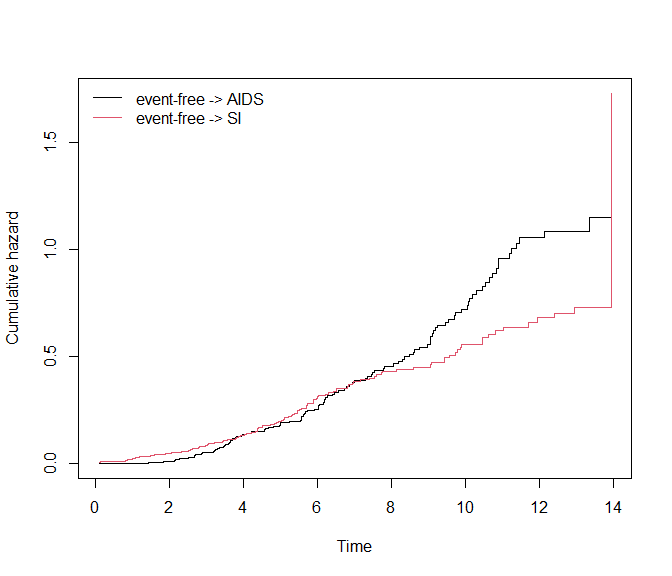If we specify the argument use.ggplot = TRUE, the plot method will return a ggplot object.

plot(msf.WW, use.ggplot = TRUE)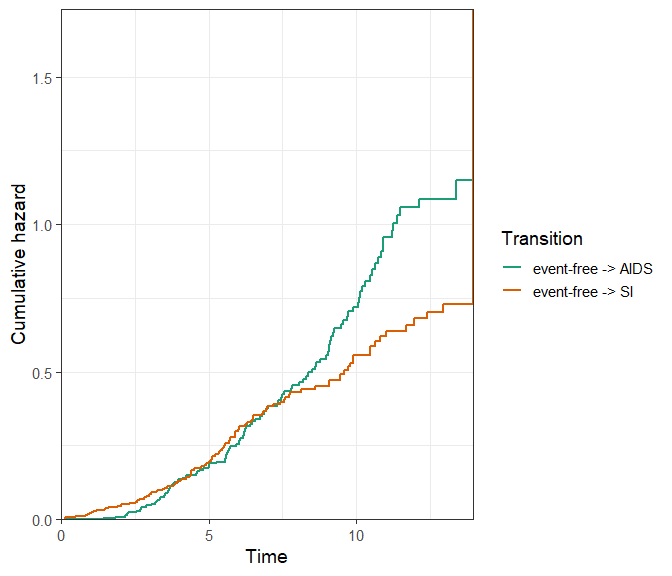When using the argument type = "separate", the base R plot will return a separate plot for each transition:

par(mfrow = c(1, 2))
plot(msf.WW, type = "separate")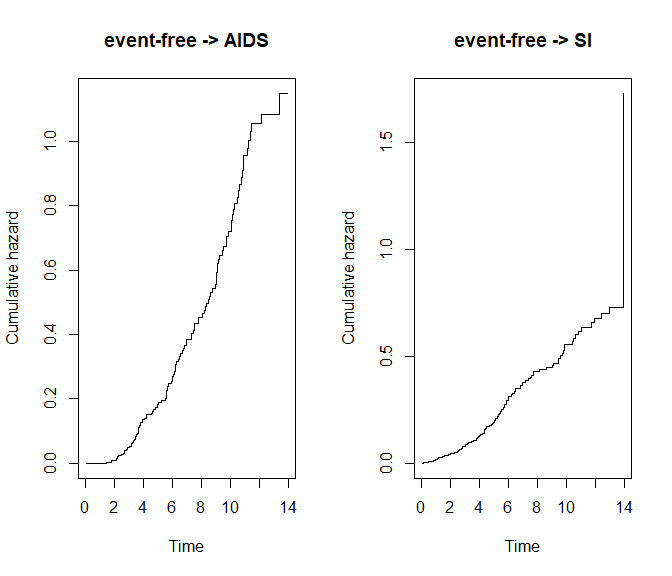The ggplot2 version will return a facetted plot, where the axis scales can either be kept “fixed,” or “free” using the scale_type argument. It is essentially the same argument as scales from the facet_wrap() function of ggplot2, see ?ggplot2::facet_wrap.

# Fixed scales
plot(msf.WW, type = "separate", use.ggplot = TRUE, scale_type = "fixed")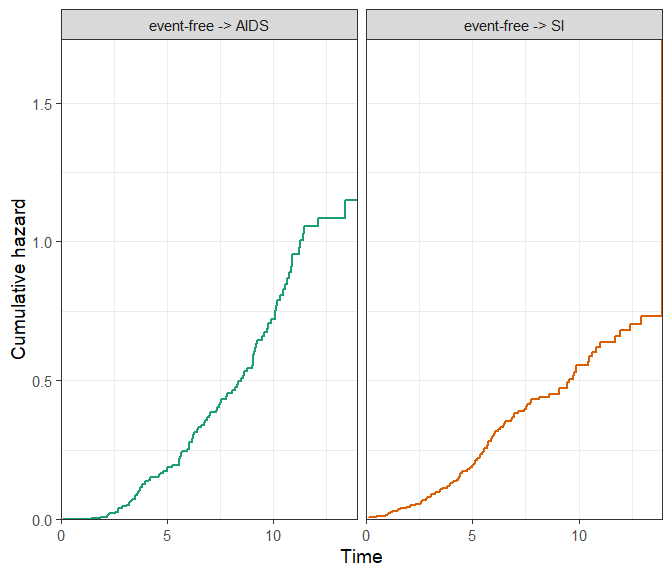# Free scales
plot(msf.WW, type = "separate", use.ggplot = TRUE, scale_type = "free", xlim = c(0, 15))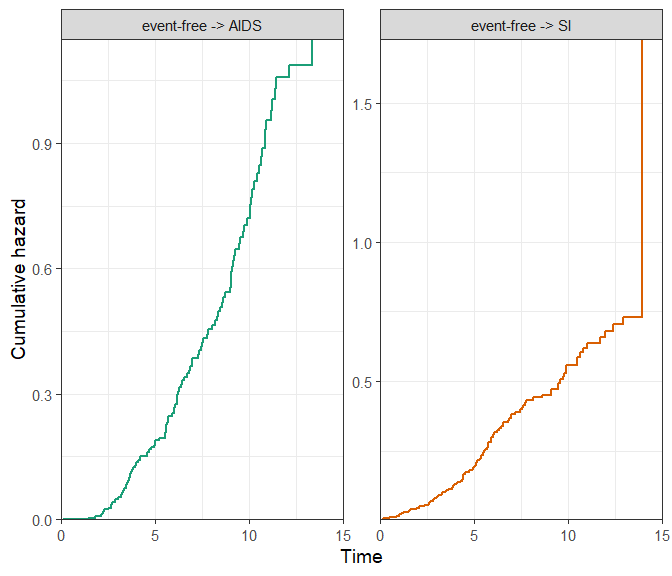The remaining arguments are standard plotting adjustments, which will work for both the ggplot2 and base R version of the plots. For details, see ?mstate::plot.msfit. Any further adjustments that are not available through the function arguments (such as plot title) can simply be added using standard ggplot2 syntax using +, or graphics functions such as title(). The following is a customised example:

par(mfrow = c(1, 1))
# A base R customised plot
plot(
msf.WW,
type = "single",
cols = c("blue", "black"), # or numeric e.g. c(1, 2)
xlim = c(0, 15),
legend.pos = "top",
lty = c("dashed", "solid"),
use.ggplot = FALSE
)
title("Cumulative baseline hazards")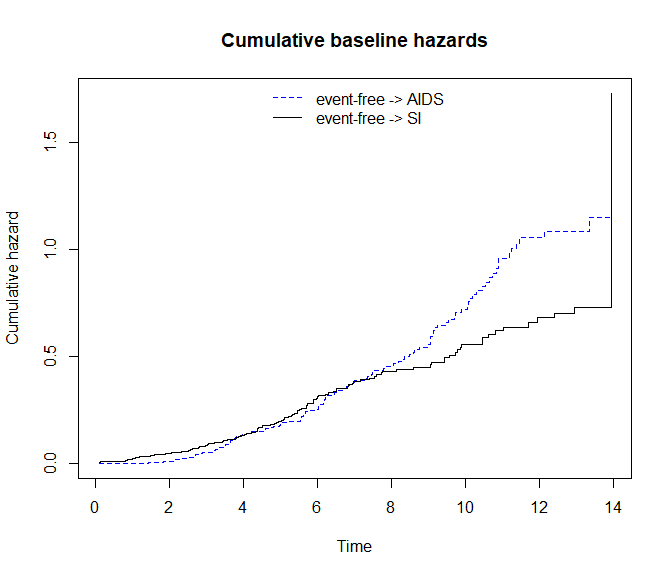# A ggplot2 customised plot
plot(
msf.WW,
type = "single",
cols = c("blue", "black"), # or numeric e.g. c(1, 2)
xlim = c(0, 15),
lty = c("dashed", "solid"),
legend.pos = "bottom",
use.ggplot = TRUE
) +

# Add title and center
ggtitle("Cumulative baseline hazards") +
theme(plot.title = element_text(hjust = 0.5))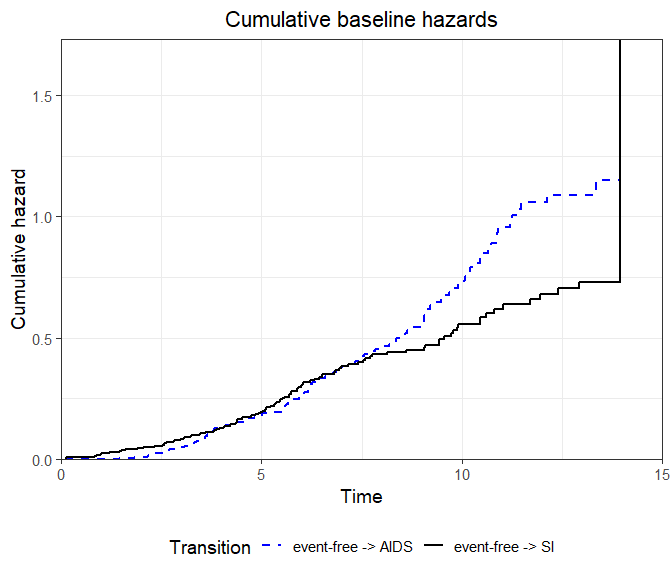Available using use.ggplot = TRUE are the confidence intervals around the cumulative hazards, which can be obtained by specifying conf.type of type “plain” or “log,” for example in single plot:

plot(
msf.WW,
type = "single",
use.ggplot = TRUE,
conf.type = "log",
conf.int = 0.95
)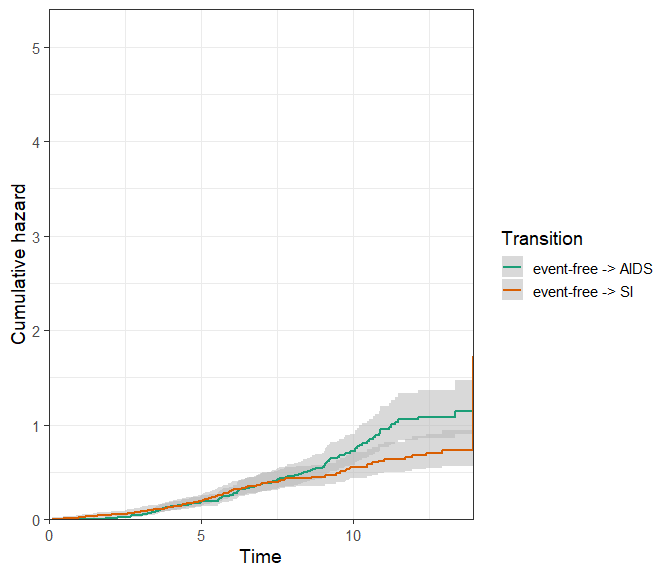Or else, in a facetted plot:

plot(
msf.WW,
type = "separate",
use.ggplot = TRUE,
conf.type = "log",
conf.int = 0.95,
scale_type = "free_y"
)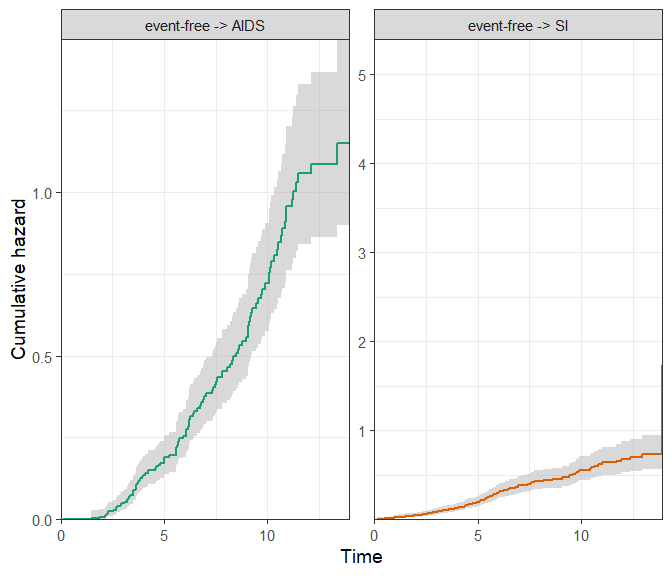# Visualising transition probabilities using plot.probtrans()

The transition hazards obtained in the previous section can be used to obtain patient-specific transition probabilities using probtrans(). Here, we would like to predict from the beginning of follow-up (predt = 0).

# Run probtrans
pt.WW <- probtrans(msf.WW, predt = 0)

# Example predict at different times
summary(pt.WW, times = c(1, 5, 10))
#>
#> Prediction from state 1 :
#>   times   pstate1   pstate2    pstate3         se1        se2         se3
#> 1     1 0.9805172 0.0000000 0.01948279 0.007899257 0.00000000 0.007899257
#> 2     5 0.6887491 0.1471596 0.16409130 0.028250825 0.02189893 0.022200323
#> 3    10 0.2772999 0.3870165 0.33568358 0.030015241 0.03202897 0.030204083
#>      lower1    lower2      lower3    upper1    upper2     upper3
#> 1 0.9651565 0.0000000 0.008801028 0.9961223 0.0000000 0.04312897
#> 2 0.6355458 0.1099311 0.125870614 0.7464063 0.1969956 0.21391771
#> 3 0.2242925 0.3290677 0.281410843 0.3428346 0.4551702 0.40042333

The plot method for probtrans objects allows to visualise the transition probabilities in various ways, using both functionality from base R graphics and ggplot2.

## Filled/stacked plots

The default is a so-called “filled” plot, where the transition probabilities are represented by coloured areas. The from argument allows the user to choose the state to predict from (default is 1, the first state).

plot(pt.WW, from = 1)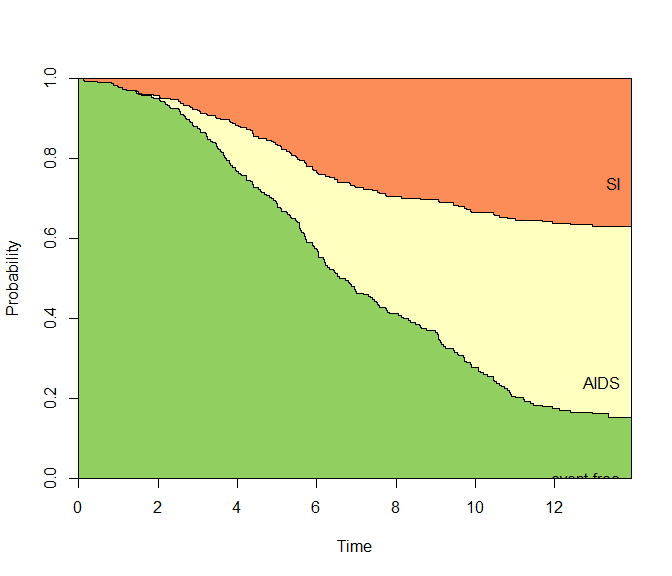Again, the use.ggplot = TRUE argument can be used to return a ggplot object instead:

# from = 1 implied
plot(pt.WW, use.ggplot = TRUE)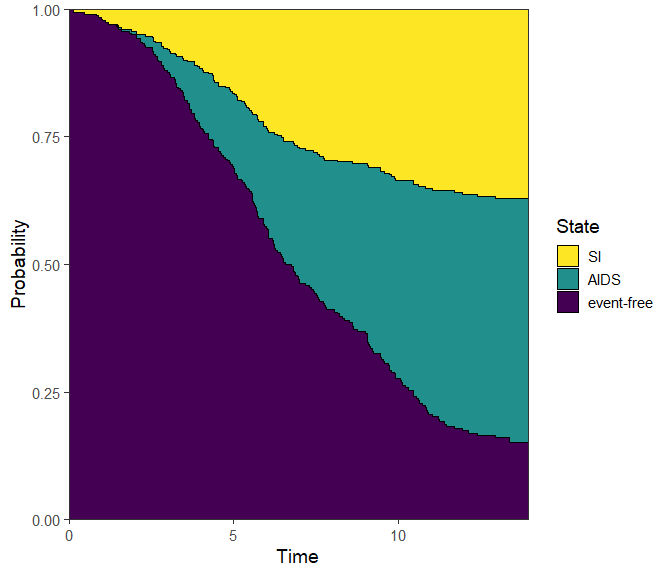Note that the ggplot2 version of the plot places the state labels in a legend rather than labels in the plot itself. If we prefer the latter, we can specify label = annotate instead - and change the size of the labels with cex.

plot(pt.WW, use.ggplot = TRUE, label = "annotate", cex = 6)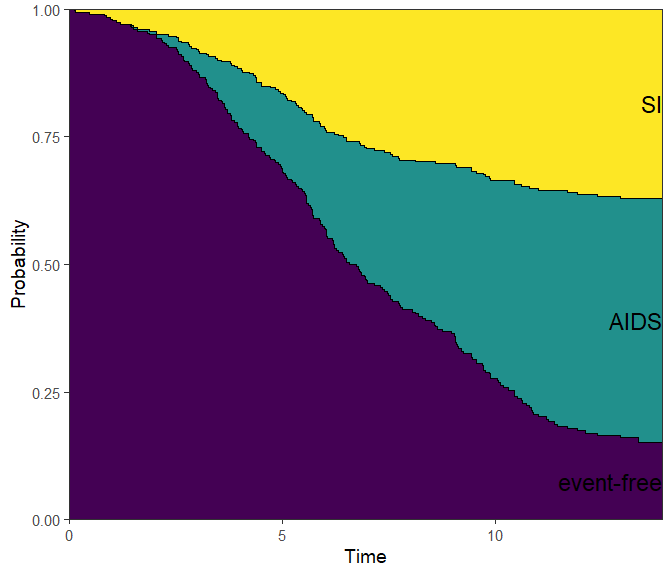More generally, we can also adjust the stacking order using the ord argument, which takes a numeric vector equal to the number of states, in the desired stacking order (bottom to top).

# Check state order again from transition matrix
tmat
#>             to
#> from         event-free AIDS SI
#>   event-free         NA    1  2
#>   AIDS               NA   NA NA
#>   SI                 NA   NA NA

# Plot aids (state 2), then event-free (state 1), and SI on top (state 3)
plot(pt.WW, use.ggplot = TRUE, ord = c(2, 1, 3))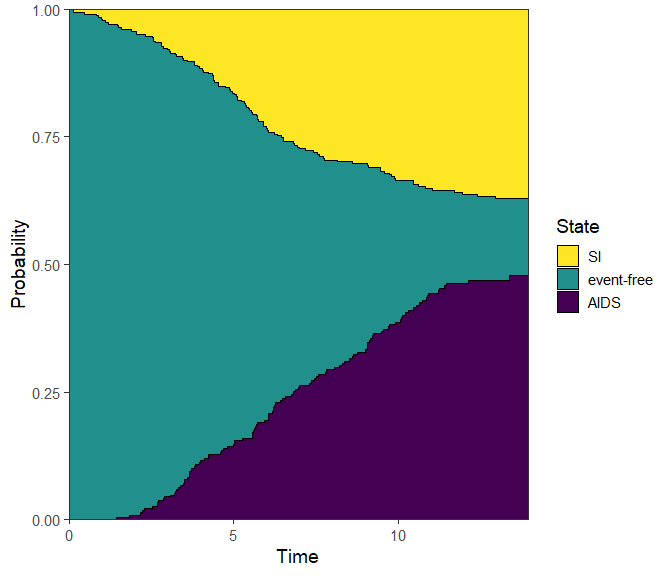You can also choose to forgo the colour, and specify type = "stacked" instead:

plot(pt.WW, use.ggplot = TRUE, type = "stacked")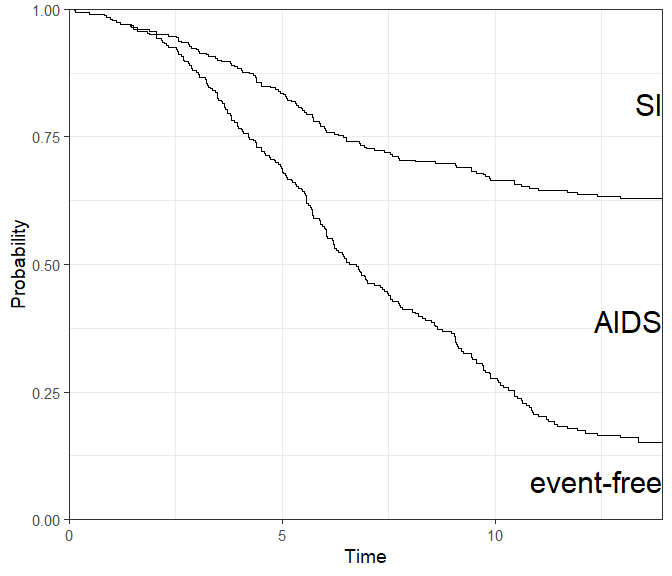## Single and separate plots, confidence intervals

Instead of visualising the probabilities using stacked areas, we can go the traditional route and use a single line per state. Confidence intervals can then be added.

By specifying type = "separate", the base R plot will return a separate plot for all three states.

par(mfrow = c(1, 3))
plot(pt.WW, type = "separate")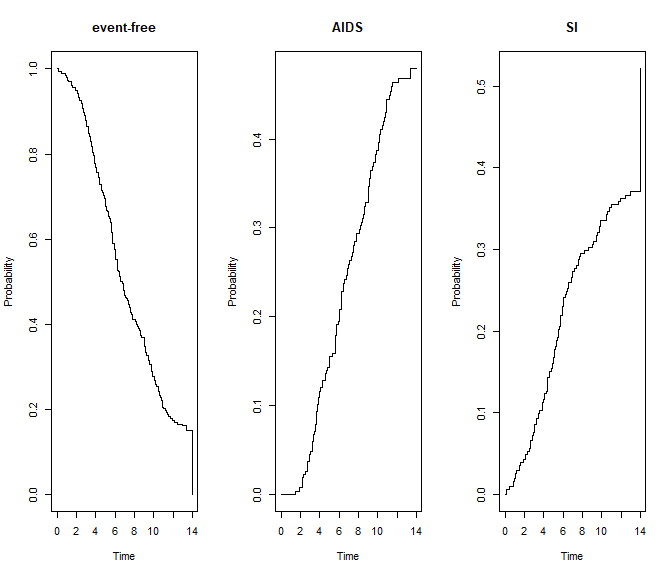The ggplot2 version will return a facetted plot, with one state per facet. It also accommodates confidence intervals, which are either of type “log” (default) or “plain.”

plot(
pt.WW,
use.ggplot = TRUE,
type = "separate",
conf.int = 0.95, # 95% level
conf.type = "log"
)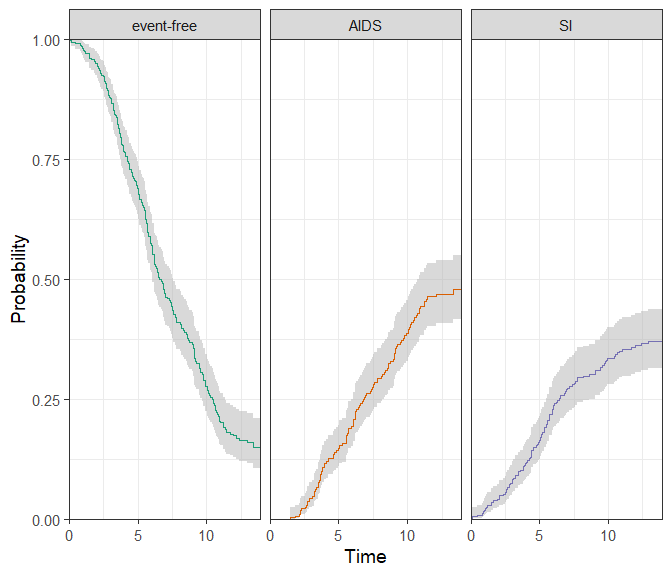These confidence intervals can be omitted using conf.type = "none".

What if we wanted all of these in one plot? For that, we can use the type = single option:

plot(
pt.WW,
use.ggplot = TRUE,
type = "single",
conf.int = 0.95, # 95% level
conf.type = "log"
)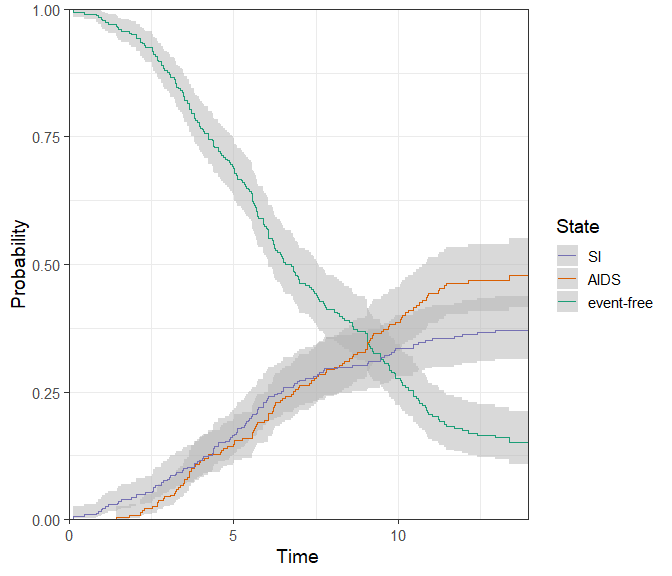As before, the confidence intervals can be omitted.

plot(
pt.WW,
use.ggplot = TRUE,
type = "single",
conf.type = "none",
lty = c(1, 2, 3), # change the linetype
lwd = 1.5,
)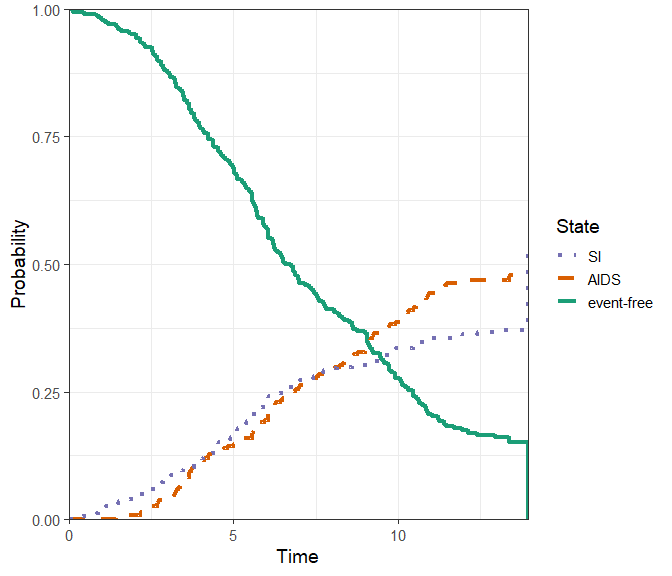If the multi-state model is large, we may be only interested in plotting the probabilities for a subset of states together. This subset will not sum up to 1, so the stacked/filled plots are not appropriate. There is no set function to do this, but it can be done by extracting the data from a plot.probtrans()-based ggplot object as follows:

# Run plot and extract data using $data dat_plot <- plot(x = pt.WW, use.ggplot = TRUE, type = "single")$data

# Begin new plot - Exclude or select states to be plotted
ggplot(data = dat_plot[state != "event-free", ], aes(
x = time,
y = prob,
ymin = CI_low,
ymax = CI_upp,
group = state,
linetype = state,
col = state
)) +

# Add CI and lines; change fill = NA to remove CIs
geom_ribbon(col = NA, fill = "gray", alpha = 0.5) +
geom_line() +

# Remaining details
labs(x = "Time", y = "Cumulative Incidence") +
coord_cartesian(ylim = c(0, 1), xlim = c(0, 14), expand = 0)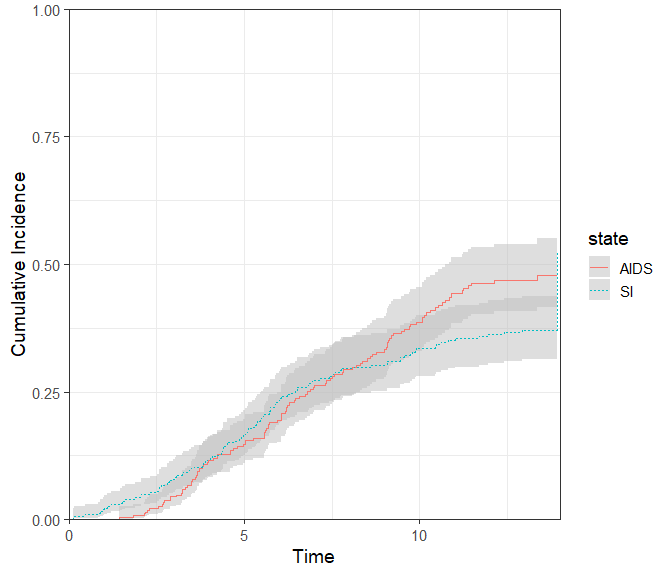If interest lies in plotting the probability of a single state, the procedure above can be used, or the vis.multiple.pt() function presented further could be used directly.

# Plotting non-parametric cumulative incidence functions

The Cuminc() function in mstate produces (for competing risks data) the non-parametric Aalen-Johansen estimates of the cumulative incidence functions. This is the same as is obtained in the cmrpsk package with the function cuminc().

In mstate, an object of class “Cuminc” can also be plotted as follows:

cum_incid <- Cuminc(
time = "time",
status = "status",
data = si
)

plot(
x = cum_incid,
use.ggplot = TRUE,
conf.type = "log",
lty = 1:2,
conf.int = 0.95,
)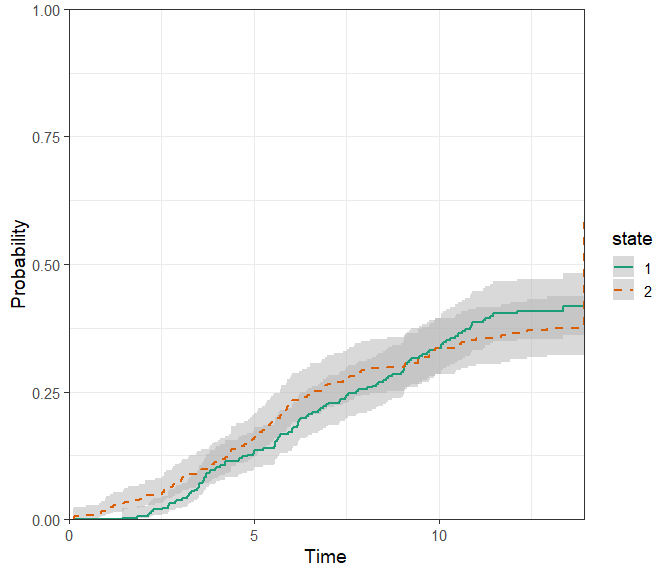In the case where this a grouping variable:

cum_incid_grp <- Cuminc(
time = "time",
status = "status",
group = "ccr5",
data = si
)

plot(
x = cum_incid_grp,
use.ggplot = TRUE,
conf.type = "none",
lty = 1:4,
facet = FALSE
)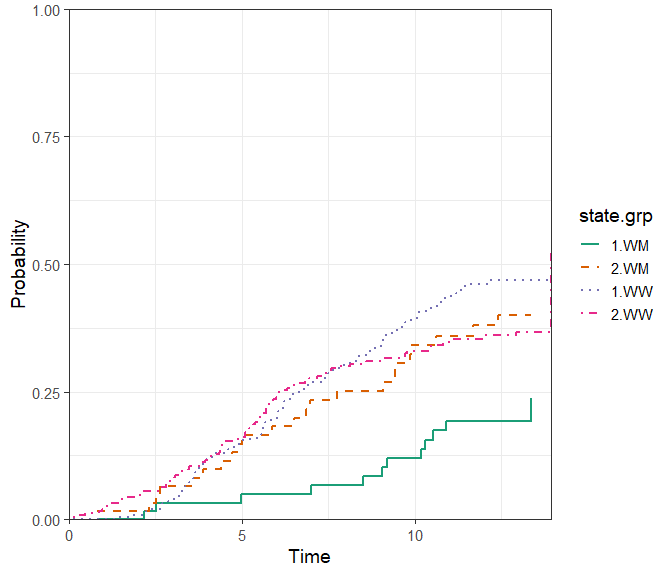plot(
x = cum_incid_grp,
use.ggplot = TRUE,
conf.type = "none",
lty = 1:4,
facet = TRUE
)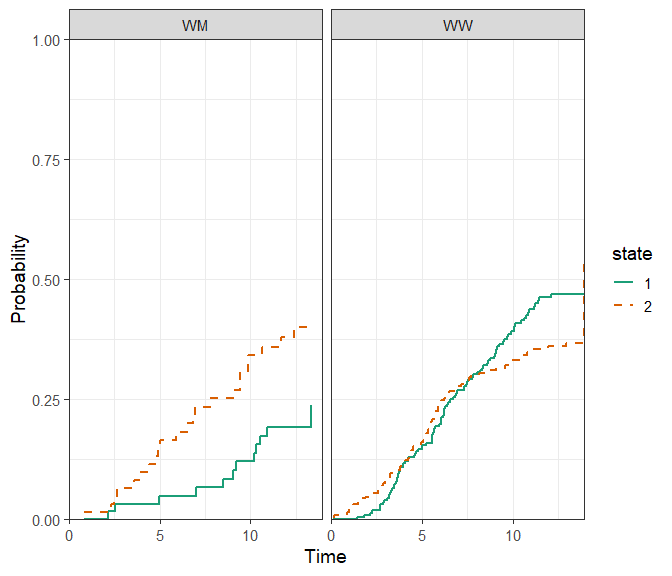# Visualising multiple probtrans objects

We may also be interested in comparing the predicted probabilities for multiple reference patients. First, we need to create as many msfit/probtrans objects as there are reference patients of interest:

# 1. Prepare patient data - both CCR5 genotypes
WW <- data.frame(
ccr5WM.1 = c(0, 0),
ccr5WM.2 = c(0, 0),
trans = c(1, 2),
strata = c(1, 2)
)

WM <- data.frame(
ccr5WM.1 = c(1, 0),
ccr5WM.2 = c(0, 1),
trans = c(1, 2),
strata = c(1, 2)
)

# 2. Make msfit objects
msf.WW <- msfit(c1, WW, trans = tmat)
msf.WM <- msfit(c1, WM, trans = tmat)

# 3. Make probtrans objects
pt.WW <- probtrans(msf.WW, predt = 0)
pt.WM <- probtrans(msf.WM, predt = 0)

Afterwards, we can supply the probtrans objects in a list into the vis.multiple.pt() function. This will visualise the probability of being in state number to over time, given the reference patient was in state number from at the predt time supplied in probtrans().

The example below visualises the probability of being in state AIDS, given event-free at time 0. The two lines/associated confidence intervals correspond to the reference patients - both with a different CCR5 genotype (“WW” or “WM”).

vis.multiple.pt(
x = list(pt.WW, pt.WM),
from = 1,
to = 2,
conf.type = "log",
cols = c(1, 2),
labels = c("Pat WW", "Pat WM"),
legend.title = "Ref patients"
)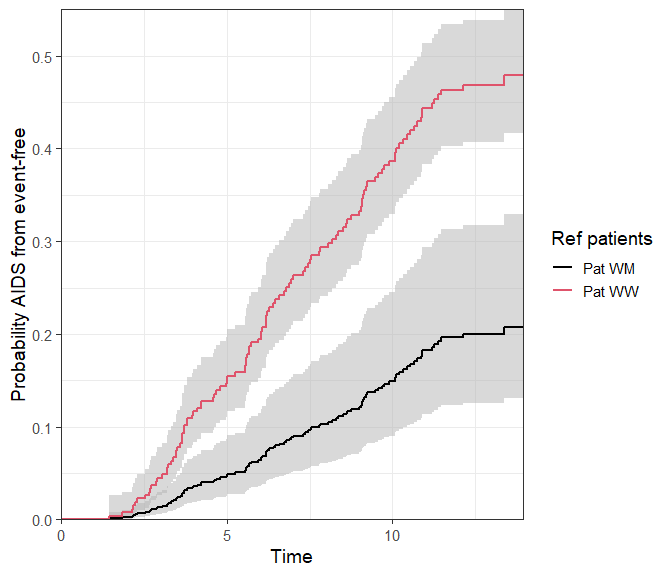This function could just as well be used for a single probtrans object:

vis.multiple.pt(
x = list(pt.WW),
from = 1,
to = 2,
conf.type = "log",
cols = c(1, 2),
labels = c("Pat WW", "Pat WM"),
legend.title = "Ref patients"
)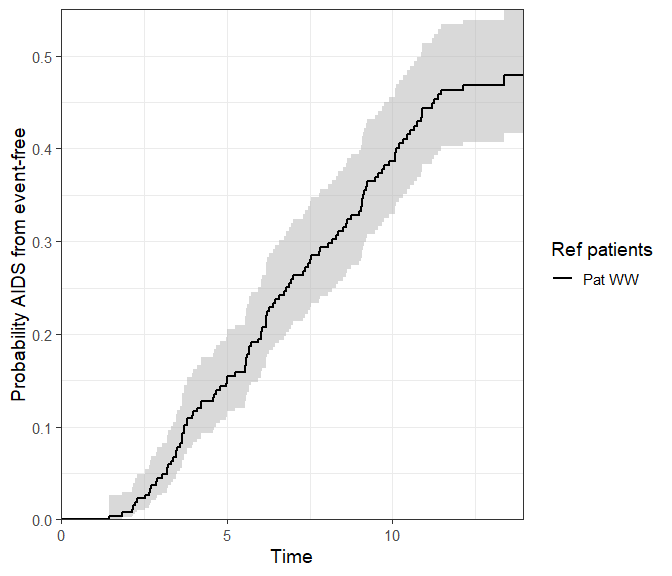Note this function is only available in the ggplot format.

## A mirrored plot

Multiple probtrans objects can also be compared by means of a mirrored plot. The idea is to compare the probabilities of being in (all) states between two references patients at a particular horizon. In addition to reference patients, different subsets of the data could equally be compared.

vis.mirror.pt(
x = list(pt.WW, pt.WM),
titles = c("WW", "WM"),
horizon = 10
)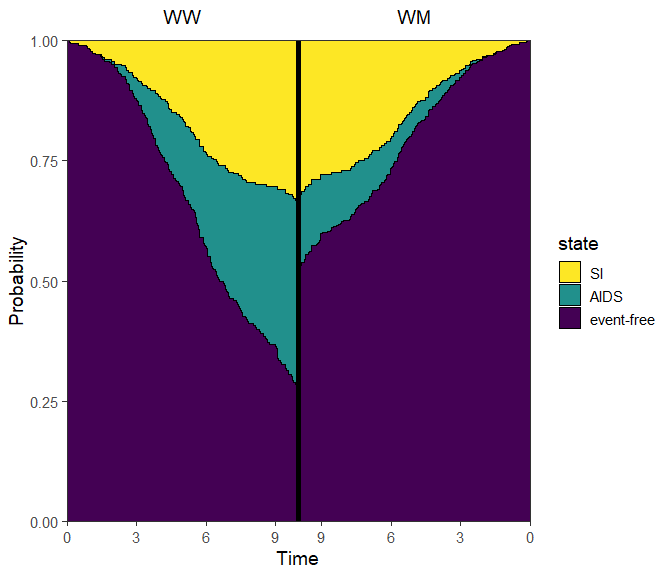Omitting the horizon argument will default to plotting both sides with their respective maximum follow-up time, so it may be asymmetric. We thus recommend to always use the horizon argument.

If for example time is in years, and follow-up is extremely short, you may want to override the breaks on the x-axis. This can be done using the breaks_x_left and breaks_x_right arguments.

vis.mirror.pt(
x = list(pt.WW, pt.WM),
titles = c("WW", "WM"),
size_titles = 8,
horizon = 5,
breaks_x_left = c(0, 1, 2, 3, 4, 5),
breaks_x_right = c(0, 1, 2, 3, 4),
ord = c(3, 2, 1)
)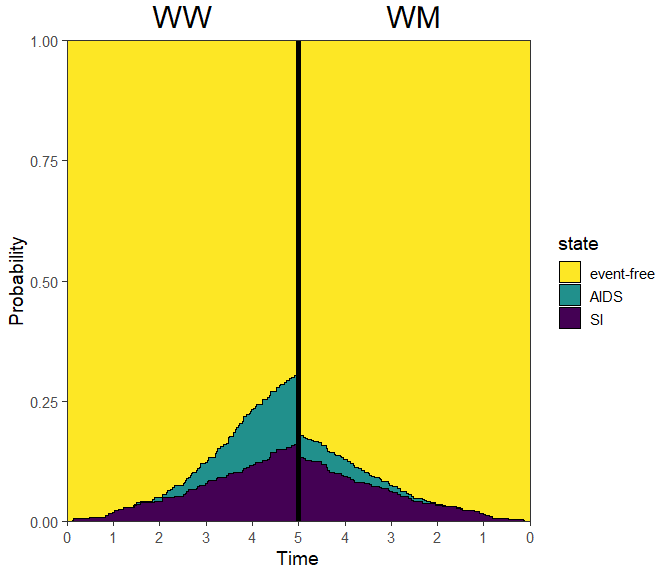# Saving outputs

Any plots made with mstate using the use.ggplot = TRUE will return a ggplot object, which can be saved to a desired format by using ggplot2::ggsave(). Please refer to the article written by the ggplot2 team on using ggplot2::ggsave().

# Saving a ggplot2 plot
p <- plot(pt.WW, use.ggplot = TRUE)
ggplot2::ggsave("my_ggplot2_plot.png")

# Standard graphics plot
png("my_standard_plot.png")
plot(pt.WW, use.ggplot = FALSE)
dev.off()

# Reproducibility

Reproducibility receipt
# Date/time
Sys.time()
#>  "2021-11-08 11:25:45 CET"

# Environment
sessionInfo()
#> R version 4.0.4 (2021-02-15)
#> Platform: x86_64-w64-mingw32/x64 (64-bit)
#> Running under: Windows 10 x64 (build 18363)
#>
#> Matrix products: default
#>
#> locale:
#>  LC_COLLATE=C                       LC_CTYPE=Dutch_Netherlands.1252
#>  LC_MONETARY=Dutch_Netherlands.1252 LC_NUMERIC=C
#>  LC_TIME=Dutch_Netherlands.1252
#>
#> attached base packages:
#>  stats     graphics  grDevices utils     datasets  methods   base
#>
#> other attached packages:
#>  ggplot2_3.3.1   mstate_0.3.2    survival_3.1-12
#>
#> loaded via a namespace (and not attached):
#>   compiler_4.0.4     pillar_1.4.4       RColorBrewer_1.1-2 highr_0.8
#>   tools_4.0.4        digest_0.6.27      viridisLite_0.3.0  evaluate_0.14
#>   lifecycle_0.2.0    tibble_3.0.1       gtable_0.3.0       lattice_0.20-41
#>  pkgconfig_2.0.3    rlang_0.4.10       Matrix_1.3-2       yaml_2.2.1
#>  xfun_0.21          withr_2.2.0        stringr_1.4.0      dplyr_1.0.0
#>  knitr_1.31         generics_0.0.2     vctrs_0.3.6        grid_4.0.4
#>  tidyselect_1.1.0   glue_1.4.2         data.table_1.12.8  R6_2.5.0
#>  rmarkdown_2.7      purrr_0.3.4        farver_2.0.3       magrittr_2.0.1
#>  scales_1.1.1       ellipsis_0.3.1     htmltools_0.5.1.1  splines_4.0.4
#>  colorspace_1.4-1   labeling_0.3       stringi_1.5.3      munsell_0.5.0
#>  crayon_1.3.4